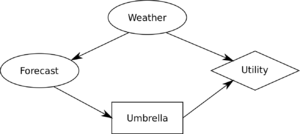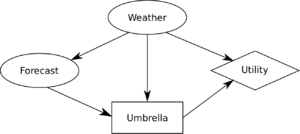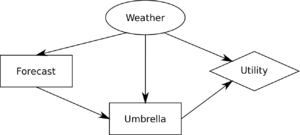# Course:CPSC522/Value of Information and Control

Jump to navigation Jump to search

## Value of Information and Control

The values of information and control quantify how access to the value of a variable, or the ability to control it, can improve an agent's utility.

Principal Author: William Harvey
Collaborators:

## Abstract

Information value theory provides a basis for evaluating actions which may provide little or no direct reward, but which provide an agent with information. Since later actions can be improved by conditioning on this information, these information-seeking actions can have indirect value, and therefore can be part of an optimal strategy. By quantifying this `value', it is possible for the agent to make comparisons between information-seeking actions and any other actions, and so identify the optimal strategy. Unlike the information theory developed by Shannon, which evaluates informativeness based purely on the probability distributions, information value theory also accounts for how the information will affect later decisions and rewards, which is necessary for determining an optimal strategy. Correspondingly, control value theory quantifies how beneficial it can be to control random variables. This control may be used to directly affect the utility, or to gain more information.

### Builds on

We discuss these concepts in the context of decision networks, which build on Bayesian networks.

### Related Pages

Quantifying the value of information has applications for decision making under uncertainty (e.g. in Partially Observable Markov Decision Processes and reinforcement learning) and for allocating sensors or processing (see bounded rationality).

## Value of Information

Access to information can have a clear value by allowing agents to make better decisions. The value of the information depends on how much better it can make the decisions: the increase in expected utility when decisions are conditioned on the information. By quantifying this value, the agent is able to actively choose to seek information, allowing a higher expected utility. For example, consider betting on a sports match: if you knew the outcome in advance, you could place a bet and be certain to make a profit. However, if you couldn't predict the outcome, the optimal strategy is likely to be not to make a bet at all. The value of this information then corresponds to the most that you would be prepared to pay to a fortune teller/match fixer to tell you the outcome.

We will now consider a more precise definition involving decision networks.

### Definition

A distinction is made between the value of perfect information (also known as the value of clairvoyance), and the value of imperfect information. Consider a non-forgetting decision network, $N$, which includes a decision variable, $D$, and a random variable, $X$. The value of perfect information about $X$for $D$is defined as the difference between:

• the value of the decision network when an arc is added from $X$to $D$(and from $X$to all later decision variables such that $N$remains a non-forgetting network)
• the value of the decision network without an arc from $X$to $D$This leads to an alternative (but equivalent) definition: the value of perfect information of $X$about $D$is the upper bound on the price that a rational decision-maker would be willing to pay to know $X$before making decision $D$.

The value of imperfect information for $X$is defined as the value of the information gained from a noisy measurement of $X$.

### Characteristics

1. The value of information is always non-negative because, in the worst case, an agent can ignore the information and so the value would be 0. Therefore, acquiring information is never harmful for a rational agent.
2. Additionally, the value of perfect information is always greater than the value of imperfect information. This means that the value of perfect information can be used to provide an upper bound on the value of imperfect information. One could use this to, for example, rule out buying a noisy sensor of $X$(which would provide imperfect information about $X$) if it would cost more than the value of perfect information about $X$.
3. The value of information about $X$is only non-zero if decisions made depend on the value of $X$.

### Example

Consider the decision networks in Figures 1-4, based on  and explained in detail in decision networks. A decision, Umbrella, must be made, determining whether or not to bring an umbrella. This should be done to maximise utility, which depends on both Weather and Umbrella, as shown in the table below:

Weather Umbrella Utility
no rain take it 20
no rain leave it 100
rain take it 70
rain leave it 0

The joint distribution over the weather and the weather forecast is shown below. It can be seen that the forecast provides some information about the weather, but not perfect information as, for example, Forecast can be rain when Weather is not rain.

Weather Forecast Probability
no rain sunny 0.49
no rain cloudy 0.14
no rain rainy 0.07
rain sunny 0.045
rain cloudy 0.075
rain rainy 0.18

#### Value with no Information

In the Figure 1, the decision must be made without knowledge about Weather or Forecast. The value for each possible decision (the expectation of the utility) is shown below:

Umbrella Value
leave it 70
take it 35

So the optimal decision is to leave the umbrella, leading to an expected utility of 70.

#### Value with Observed ForecastFigure 2. A decision, Umbrella, must be made conditioned on a weather forecast, Forecast. The utility depends on both Umbrella and Weather, the true weather. Based on Section 9.3.3 of "Artificial Intelligence: Foundations of Computational Agents"

In Figure 2, the decision can depend on Forecast. As shown in , this gives the following decisions are optimal for each value of Forecast:

Forecast Umbrella Value
sunny leave it 91.6
cloudy leave it 65.1
rainy take it 56

Taking an expectation over the values of Forecast, the expected utility is 77. This is 7 greater than the value without conditioning on the Weather, and so the information value of Forecast for Umbrella is 7. Note that, since Forecast is essentially a noisy measurement of Weather, this can be viewed as the value of perfect information about Forecast or, alternatively, as the value of imperfect information about Weather. Also, note that it is positive, showing that information about the weather forecast improves decision-making about bringing an umbrella (and satisfying the requirement that the value of information is non-negative).

#### Value with Observed WeatherFigure 3. A decision, Umbrella, must be made conditioned on perfect information about the weather. The utility depends on both Umbrella and Weather, the true weather. Based on Section 9.3.3 of "Artificial Intelligence: Foundations of Computational Agents"

Now consider Figure 3. In this, the agent is able to condition its decision on the true value of Weather, rather than relying on the imperfect information contained by Forecast. This leads to the decisions shown below:

Weather Umbrella Value
no rain leave it 100
rain take it 70

Taking an expectation over Weather reveals that the value is 91. This is 21 greater than without information, and so the value of perfect information about Weather is 21. This is greater than the value of the imperfect information about Weather we considered previously (ie the perfect information about Forecast), satisfying the characteristic that the value of perfect information is an upper bound on the value of imperfect information.

#### Value with Observed Weather and ForecastFigure 4. A decision, Umbrella, must be made conditioned on perfect information about both the weather and the weather forecast. The utility depends on Umbrella and Weather, the true weather. Based on Section 9.3.3 of "Artificial Intelligence: Foundations of Computational Agents"

Now consider Figure 4, in which the agent has access to both Weather and Forecast when making the decision. This leads to the decisions and values shown below:

Weather Forecast Umbrella Value
no rain sunny leave it 100
no rain cloudy leave it 100
no rain rainy leave it 100
rain sunny take it 70
rain cloudy take it 70
rain rainy take it 70

Taking an expectation over Weather and Forecast gives a value of 91. This is identical to the situation where we observed only Weather. This can be explained by the fact that, in this case, we already had perfect information about Weather. Since Forecast is simply a noisy measurement of Weather, and does not otherwise affect the utility, information about it is redundant when we already know Weather. Therefore, we can say that the value of information about Forecast is zero when there is an arc from Weather to Umbrella. Note that, as with any random variable with a value of zero, the decisions made do not depend on the value of Forecast (see the table above).

### Sequences of Decisions

The example above dealt with a decision network with a single decision variable. The value of information theory can also be applied when decisions are made sequentially. In this case, there is the added complication that the time when we obtain the information becomes important. For example, it is important to have information about the weather before deciding whether or not to take an umbrella, even if more decisions will be made later. Therefore, the value of information can be different depending on when it is obtained.

## Value of ControlFigure 5. Decision network with Forecast turned into a decision variable. It still has the same parent as before. An additional arc has been drawn from the parent, Weather, to Umbrella, to ensure that the network remains non-forgetting. Based on Section 9.3.3 of "Artificial Intelligence: Foundations of Computational Agents"

Similarly to the value of information quantifying the value of knowledge about a random variable, the value of control quantifies the value in being able to control it. In the context of decision networks, the value of control is defined as the increase in value when a random variable is replaced with a decision node and edges are added to make it a no-forgetting network (so that later decision variables have access to all random variables that previous decision variables had access to - see the page on decision networks). The properties of the new network depend on which nodes are used as parents to the new decision variable. We now discuss two possible cases:

### Control with Parents Maintained

This means that the decision can be made using all the information that the random variable could depend on. In the context of decision networks, the decision variable has the same parents as the random variable it replaces. In this case, controlling the variable may also involve adding edges from the parents to later decision variables to maintain the no-forgetting property.

When the parents are maintained, the value of control is always non-negative, and no less than the value of perfect information about the same node.

#### Example

Consider the decision network in Figure 4. It is based on the example from the page on decision networks but Forecast has been turned into a decision node. Its parent nodes are the same as before, and an additional arc has been drawn from Weather to Umbrella to ensure that this is a no-forgetting network. This additional arc ensures that the decision node, Umbrella, has perfect information about the weather, and so the value is 91 - meaning that the value of control in this context is 14. As with any case where the parents are maintained, the value of control of Forecast (14) proves to be no less than the value of perfect information (0, since Forecast was already a parent of Umbrella). Note that, although, the value of control of Forecast is equal to the value of perfect information about Weather in this case, there is not always such a correspondence. For example, if Weather was controlled, it could always be set to not rain and the value of control would be 30, greater than the value of perfect information about any node.

### Control with Different Parents

If the decision variable does not have all of the same parents as the random variable, the value of control can be less than the value of information and can, in fact, be negative.

#### Example

As an example, consider the decision network in Figure 5, where Forecast has been turned into a decision variable with no parents. Forecast is now controlled, but cannot depend on Weather. Therefore, Umbrella cannot depend on Weather either and so the value is 70. Since the value of the decision network was 77 before Weather was controlled, the value of control of Weather is -7. This is negative because Umbrella has gone from having imperfect information about Weather to having no information, whilst controlling Forecast cannot increase the utility on its own. The fact that the value of control is negative illustrates that control is not necessarily helpful if the parents change, and shows the importance of specifying the parents of controlled variables when evaluating the value of control.

## Annotated Bibliography

1. David Poole & Alan Mackworth, "Artificial Intelligence: Foundations of Computational Agents", Chapter 9.4
2. *Howard RA. Information value theory. IEEE Transactions on systems science and cybernetics. 1966 Aug;2(1):22-6.